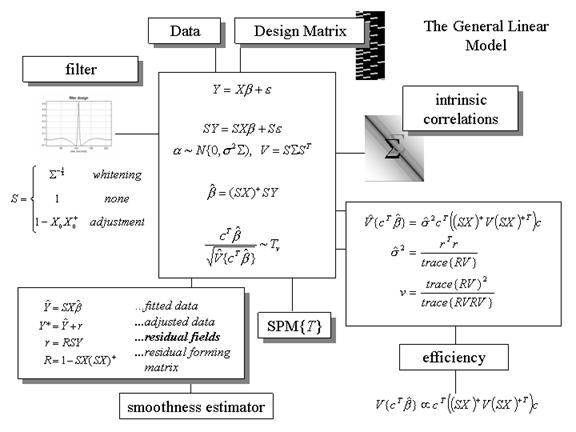Figure 4

The general linear model. The general linear model is an equation expressing the response variable Y in terms of a linear combination of explanatory variables in a design matrix X and an error term with assumed or known autocorrelation S. In fMRI the data can be filtered with a convolution or residual forming matrix (or a combination) S, leading to a generalized linear model that includes [intrinsic] serial correlations and applied [extrinsic] filtering. Different choices of S correspond to different estimation schemes as indicated on the upper left. The parameter estimates obtain in a least squares sense using the pseudoinverse (denoted by +) of the filtered design matrix. Generally an effect of interest is specified by a vector of contrast weights c that give a weighted sum or compound of parameter estimatesreferred to as a contrast. The T statistic is simply this contrast divided by its the estimated standard error (i.e. square root of its estimated variance). The ensuing T statistic is distributed withv degrees of freedom. The equations for estimating the variance of the contrast and the degrees of freedom associated with the error variance are provided in the right-hand panel. Efficiency is simply the inverse of the variance of the contrast. These expressions are useful when assessing the relative efficiency of experimental designs. The parameter estimates can either be examined directly or used to compute the fitted responses (see lower left panel). Adjusted data refers to data from which estimated confounds have been removed. The residuals r obtain from applying the residual-forming matrix R to the data. These residual fields are used to estimate the smoothness of the component fields of the SPM used in random field theory (see Figure 7.htm).It should be noted that generalised linear models are much more extensive than linear models with non-spherical Gaussian errors and cover models with non-Gaussian errors. This means that the generalised linear models referred to in this book are the simplest generalisation of general linear models.